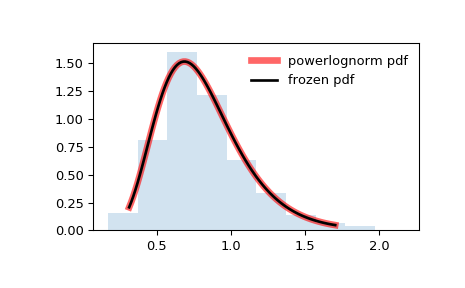# scipy.stats.powerlognorm¶

scipy.stats.powerlognorm = <scipy.stats._continuous_distns.powerlognorm_gen object>[source]

A power log-normal continuous random variable.

As an instance of the rv_continuous class, powerlognorm object inherits from it a collection of generic methods (see below for the full list), and completes them with details specific for this particular distribution.

Notes

The probability density function for powerlognorm is:

$f(x, c, s) = \frac{c}{x s} \phi(\log(x)/s) (\Phi(-\log(x)/s))^{c-1}$

where $$\phi$$ is the normal pdf, and $$\Phi$$ is the normal cdf, and $$x > 0$$, $$s, c > 0$$.

powerlognorm takes $$c$$ and $$s$$ as shape parameters.

The probability density above is defined in the “standardized” form. To shift and/or scale the distribution use the loc and scale parameters. Specifically, powerlognorm.pdf(x, c, s, loc, scale) is identically equivalent to powerlognorm.pdf(y, c, s) / scale with y = (x - loc) / scale.

Examples

>>> from scipy.stats import powerlognorm
>>> import matplotlib.pyplot as plt
>>> fig, ax = plt.subplots(1, 1)


Calculate a few first moments:

>>> c, s = 2.14, 0.446
>>> mean, var, skew, kurt = powerlognorm.stats(c, s, moments='mvsk')


Display the probability density function (pdf):

>>> x = np.linspace(powerlognorm.ppf(0.01, c, s),
...                 powerlognorm.ppf(0.99, c, s), 100)
>>> ax.plot(x, powerlognorm.pdf(x, c, s),
...        'r-', lw=5, alpha=0.6, label='powerlognorm pdf')


Alternatively, the distribution object can be called (as a function) to fix the shape, location and scale parameters. This returns a “frozen” RV object holding the given parameters fixed.

Freeze the distribution and display the frozen pdf:

>>> rv = powerlognorm(c, s)
>>> ax.plot(x, rv.pdf(x), 'k-', lw=2, label='frozen pdf')


Check accuracy of cdf and ppf:

>>> vals = powerlognorm.ppf([0.001, 0.5, 0.999], c, s)
>>> np.allclose([0.001, 0.5, 0.999], powerlognorm.cdf(vals, c, s))
True


Generate random numbers:

>>> r = powerlognorm.rvs(c, s, size=1000)


And compare the histogram:

>>> ax.hist(r, normed=True, histtype='stepfilled', alpha=0.2)
>>> ax.legend(loc='best', frameon=False)
>>> plt.show()Methods

 rvs(c, s, loc=0, scale=1, size=1, random_state=None) Random variates. pdf(x, c, s, loc=0, scale=1) Probability density function. logpdf(x, c, s, loc=0, scale=1) Log of the probability density function. cdf(x, c, s, loc=0, scale=1) Cumulative distribution function. logcdf(x, c, s, loc=0, scale=1) Log of the cumulative distribution function. sf(x, c, s, loc=0, scale=1) Survival function (also defined as 1 - cdf, but sf is sometimes more accurate). logsf(x, c, s, loc=0, scale=1) Log of the survival function. ppf(q, c, s, loc=0, scale=1) Percent point function (inverse of cdf — percentiles). isf(q, c, s, loc=0, scale=1) Inverse survival function (inverse of sf). moment(n, c, s, loc=0, scale=1) Non-central moment of order n stats(c, s, loc=0, scale=1, moments='mv') Mean(‘m’), variance(‘v’), skew(‘s’), and/or kurtosis(‘k’). entropy(c, s, loc=0, scale=1) (Differential) entropy of the RV. fit(data, c, s, loc=0, scale=1) Parameter estimates for generic data. expect(func, args=(c, s), loc=0, scale=1, lb=None, ub=None, conditional=False, **kwds) Expected value of a function (of one argument) with respect to the distribution. median(c, s, loc=0, scale=1) Median of the distribution. mean(c, s, loc=0, scale=1) Mean of the distribution. var(c, s, loc=0, scale=1) Variance of the distribution. std(c, s, loc=0, scale=1) Standard deviation of the distribution. interval(alpha, c, s, loc=0, scale=1) Endpoints of the range that contains alpha percent of the distribution

#### Previous topic

scipy.stats.powerlaw

#### Next topic

scipy.stats.powernorm